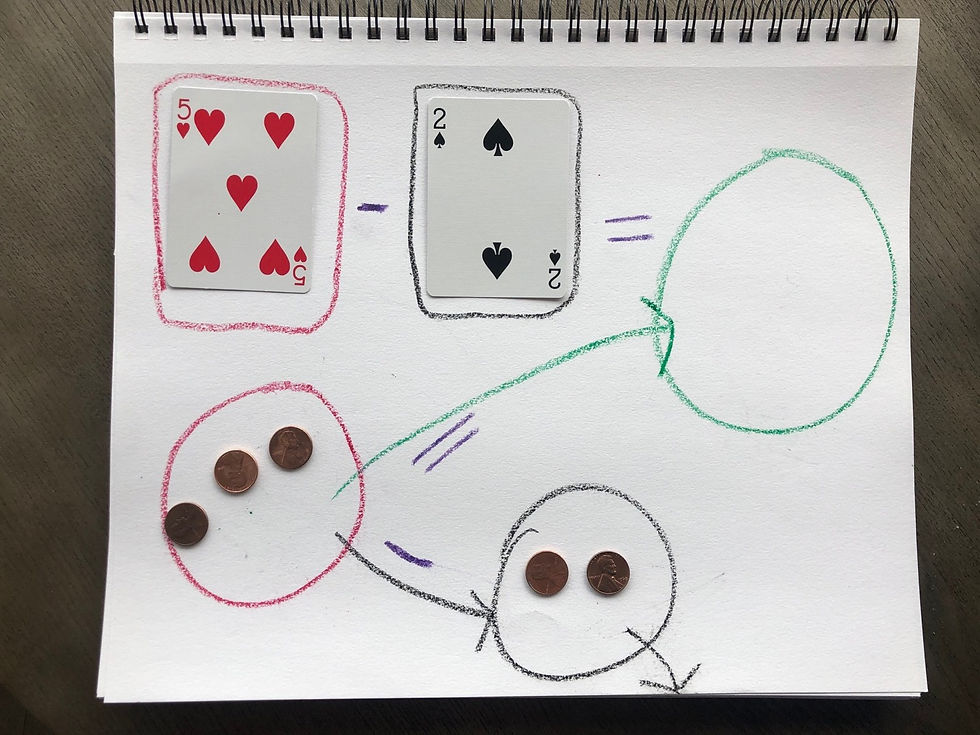top of page
Search
• Emily

# Math Lesson With Cards and CoinsLooking to do a math game with things you have on hand? This is a great way to teach addition and subtraction in a hands on way.

What you need:

• paper and crayons

• deck of cards

• pennies

Let‘s start with addition as it is the easier one to grasp. I started by setting up our paper like this to demonstrate equations - one red rectangle (playing card sized) + one black rectangle = , and then below I repeated the equation with circles. then in the equal spot I made one large circle.Also while setting up I made a pile of red cards and black cards (Ace to 10) - you will likely need to explain to your child that the Ace is the same as 1.

Now allow your child to draw one black and one red card (or if you want to keep equations small you can pick the cards yourself) and place them in the corresponding squares.

Then have them count out the same number of pennies in the circles below each card.Now have your child move the pennies from both circles into the large circle in the = spot. Then have her count the number of total pennies. Tada! Addition. I have my son say the full equation aloud for practice and as he says it, I write it down (or you can have your child do it) on a separate price of paper for a little visual reinforcement.

Subtraction is a little trickier but the same idea. This time prepare your paper with two rectangles, red and black like last time. One circle below the first card, then a smaller circle down low and a circle in the = spot.You will want to pick the cards this time so you don’t wind up with negatives. Let’s say you come up with 5 - 2. Have your child count out 5 pennies into the first circle.Then move 2 pennies from that circle into the small circle and the remaining two pennies into the = circle.For the sake of clarity I then had my kiddo move the subtracted pennies off the paper entirely and then count the remaining pennies in the = circle. Repeat the equation out loud while you or your child write it out. There ya go, subtraction!